Maths-
General
Easy

Question

# In a school,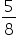𝑡ℎ of the students are boys, if there are 240 girls, find the number of boys in the school?Hint:

## The correct answer is: 400

### Let the total number of students = xNumber of girls = 240So, number of boys = x - 240…(i)Given that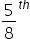of the total students are boys. That is,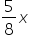…(ii)On equating (i) and (ii) , we get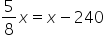Multiply by 8 on both sides.We have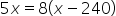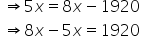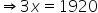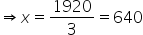Hence total number of students = 640From (ii), we get total number of boys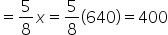So, number of boys in the school = 400#### With Turito Foundation.#### Get an Expert Advice From Turito.